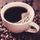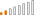cancel
Showing results for
Did you mean:Continued Contributor

## Trying to understand usage/logic of SUMX (outcome is illogical)

I am sharing my Pbix file here.

I am trying to understand about "SUMX" in general (because somebody suggested to use it), but I am not sure how it works. I got the impression that SUMX counts all occurrence/evaluation in the row context.

I decided to use "SUMX" because I have cases where I have to make the outcome as "", and if I do not use SUMX, Total comes out to be "" in Maxtrix, and that is not it should look.

I am working on measures called "Step1_0821" & "Step1 0821 SUMX".

According to the logic, it should generate 1 in both"Step1_0821" & "Step1 0821 SUMX", but on "Step1 0821 SUMX", the outcome is 2, and that is why I am trying to get a feedback from the community.If I understand the flow of logic correctly in this case, the logic did not meet the requirement for vNotEligible, so it goes to the final choice option, Step1, for the outcome of 1.

But, with the logic of Step1, it should generate only 1 row for Step1 0821 SUMX or I am writing the DAX formula correctly.

Where am I missing the logic?

1 ACCEPTED SOLUTIONSuper User

@JustinDoh1 Right, in general you either use CALCULATE or SUMX. When you mix the two you could definitely get some odd results.

Become an expert!: Enterprise DNA
External Tools: MSHGQM
Latest book!:
Mastering Power BI 2nd EditionDAX is easy, CALCULATE makes DAX hard...
6 REPLIES 6Super User

@JustinDoh1 Sorry, I don't see SUMX in your formulas? Reasons to use SUMX. SUMX accepts a table expression as the first parameter so you can filter it however you please and then SUMX takes the second parameter, evaluates the expression for each row of the table and then sums the results together. You can see the difference between SUMX and CALCULATE. Take a simple table like below:

ItemQuantityValue

 One 5 5 Two 10 10 Three 20 20

And the following measures:

``````Sumx1 = SUMX(FILTER('Items',[Item]<>"Two"),[Quantity]*[Value])

Caculate1 = CALCULATE(SUM('Items'[Quantity])*SUM('Items'[Value]),'Items'[Item]<>"Two")``````

SUMX returns the correct answer 425 while CALCULATE returns the wrong answer 625. SUMX got the right answer because it evaluated at the row level, 5*5 + 20*20. CALCULATE got it wrong because it calculated (20 + 5) * (20 + 5).

Become an expert!: Enterprise DNA
External Tools: MSHGQM
Latest book!:
Mastering Power BI 2nd EditionDAX is easy, CALCULATE makes DAX hard...Continued Contributor

@Greg_Deckler Thank you for your feedback. I was using SUMX calling measure "Step1_0821" like this:So, you suggest to use SUMX on this area directly?Super User

@JustinDoh1 Right, in general you either use CALCULATE or SUMX. When you mix the two you could definitely get some odd results.

Become an expert!: Enterprise DNA
External Tools: MSHGQM
Latest book!:
Mastering Power BI 2nd EditionDAX is easy, CALCULATE makes DAX hard...Continued Contributor

@Greg_Deckler Thank you so much. I am getting close to make this work.

When I tried with two criteria within filter, it would not give me error.

But when I tried with more than two, it would not let me, but gives me an error.

Do I go about doing this?Super User

@JustinDoh1 Your NOT(ISBLANK is missing a closing paren. Should be

NOT(ISBLANK(Table1[Consent]))

Become an expert!: Enterprise DNA
External Tools: MSHGQM
Latest book!:
Mastering Power BI 2nd EditionDAX is easy, CALCULATE makes DAX hard...Frequent Visitor

@Greg_Deckler Thank you so much. I was searching for an example online for "SUMX multiple filters", and only found examples up to 2 filters, so I lost a confidence.